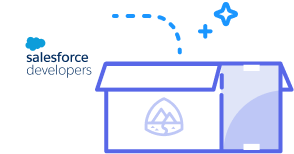function readOnly(count){ }Starting November 20, the site will be set to read-only. On December 4, 2023,
forum discussions will move to the Trailblazer Community.
ShowAll Questionssorted byDate PostedJFro

# Need help with a formula

I'm trying to update a date field Last_QCCP_Call__c using a field update with the following:

I'm getting the following error:  Error: Formula result is data type (Boolean), incompatible with expected data type (Date).﻿

IF(today() <= DATE(2011,06,01), Last_QCCP_Call__c=DATE(2011,03,01),
IF(today() <= DATE(2011,09,01), Last_QCCP_Call__c=DATE(2011,06,01),
IF(today() <= DATE(2011,12,01), Last_QCCP_Call__c=DATE(2011,09,01),
IF(today() <= DATE(2012,03,01), Last_QCCP_Call__c=DATE(2011,12,01), null
)
)
)
)﻿Best Answer chosen by Admin (Salesforce Developers)Jake Gmerek

Jfro, you are trying to create a field update correct, not a formula field on your object? (you went under setup->Create->Workflows and Approvals->Fieldupdates or similar)  If it is a field update this should work:

)﻿﻿

IF(today() <= DATE(2011,06,01), DATE(2011,03,01),
IF(today() <= DATE(2011,09,01), DATE(2011,06,01),
IF(today() <= DATE(2011,12,01), DATE(2011,09,01),
IF(today() <= DATE(2012,03,01), DATE(2011,12,01), null
)
)
)

In this case the assignment is happening automatically so you do not need to explicitly tell the formula which field to update.Shashikant Sharma

Your IF function in formula should return a date as your return type is date but it is returning a boolean , either you have to create a new field with return type boolean if you want a boolean return type or change the formula to return a date in all cases.

Like this Last_QCCP_Call__c=DATE(2011,03,01)﻿ will return a boolean not date.JFro

How would i change the formula to return a date.  I've tried so many variations....  Your help is much appreicated.Shashikant Sharma

You can not change the formula return type from date to boolean, you have to create new formula field of return type Boolean.  Even though there are some options that come for date return type formula, but no option to convert a date formula to boolean.You can delete this old one if you do not want to use it.﻿

To see what options you have : Edit your formula field -> Formula Options﻿ -> see the pick list  "Formula Return Type"JFro

A new formula in the field update?

I'm struggling to understand what you're telling me to do.  Sorry :(Shashikant Sharma

I am telling you a new formula field with return type boolean , use your formula there, instead of null in your formula pass true or falseJake Gmerek

Jfro, you are trying to create a field update correct, not a formula field on your object? (you went under setup->Create->Workflows and Approvals->Fieldupdates or similar)  If it is a field update this should work:

)﻿﻿

IF(today() <= DATE(2011,06,01), DATE(2011,03,01),
IF(today() <= DATE(2011,09,01), DATE(2011,06,01),
IF(today() <= DATE(2011,12,01), DATE(2011,09,01),
IF(today() <= DATE(2012,03,01), DATE(2011,12,01), null
)
)
)

In this case the assignment is happening automatically so you do not need to explicitly tell the formula which field to update.

This was selected as the best answerJFro

You are absolutely correct.  Thank you, thank you.  I didn't even realize I already selected the field to update.

I'm a complete Novice, what can i say.

Much appreciated.Jake GmerekJosh Gruberman 7